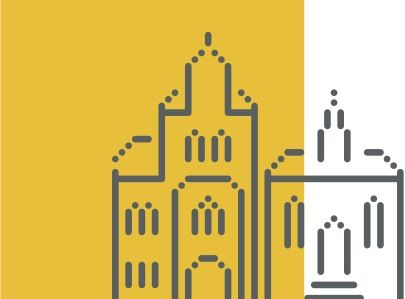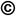TOP
##### Search the Dagstuhl Website
Looking for information on the websites of the individual seminars? - Then please:
Not found what you are looking for? - Some of our services have separate websites, each with its own search option. Please check the following list:##### Seminars
###### External resources:
• DOOR (for registering your stay at Dagstuhl)
• DOSA (for proposing future Dagstuhl Seminars or Dagstuhl Perspectives Workshops)
##### dblp
###### External resources:
• the dblp Computer Science Bibliography

### Optimal algorithms and proofs

##### Organizers
• (University of Leeds, GB)
• Edward A. Hirsch (Steklov Institute - St. Petersburg, RU)
• (Charles University - Prague, CZ)
• (University of Edinburgh, GB)

##### Motivation

The notion of optimality plays a major role in theoretical computer science. Given a computational problem, does there exist a "fastest" algorithm for it? Which proof system yields the shortest proofs of propositional tautologies? Is there a single distribution which can be used to inductively infer any computable sequence? Given a class of optimization problems, is there a single algorithm which always gives the best effcient approximation to the solution? Each of these questions is a foundational one in its area – the first in computational complexity, the second in proof complexity, the third in computational learning theory, and the last in the theory of approximation.

Consider, as an example, the Boolean Satisfiability (SAT) search problem, which asks, given a Boolean formula, for a satisfying assignment to the formula. Since SAT is NP-complete, being able to tell whether the fastest algorithm for SAT runs in polynomial time would imply a solution to the notoriously hard NP vs P problem. However, the possibility remains that we can define an optimal algorithm which we can guarantee to be essentially the fastest on every instance, even if we cannot rigorously analyze the algorithm. In a seminal paper, Leonid Levin (1973) proved that every NP search problem, and in particular SAT, has an optimal algorithm. It is still unknown whether every decision problem in NP has an optimal algorithm.

In general, given a class of computational artefacts (algorithms/proof systems/distributions) and performance measures for each artefact in the class, we say that an artefact is optimal if it matches the performance of every other arte-fact in every case. The main questions about optimality to be discussed in the seminar are: which computational models allow for optimality results, either in terms of optimal algorithms or optimal proofs? In case they do exist, how well do they match the performance of other artefacts in the class? How is the existence of optimal artefacts related to other fundamental theoretical questions, such as complexity lower bounds, effcient learnability or approximability?

There have been a number of important recent results about optimality in various computational settings. Prime examples include optimal proof systems and acceptors under advice or in heuristic settings, surprising relations of optimal proof systems to descriptive complexity and parameterized complexity, hierarchy results in various computational settings, and optimal approximation algorithms for constraint satisfaction problems.

Given these advances it is the right time for a seminar to bring together researchers working on similar questions in different areas, for an exchange of ideas and techniques. Although researchers on optimality share some common methodology – for instance, Kolmogorov complexity – each research community among our prime target areas of proof complexity, logic, computational complexity, and approximations has its own set of techniques and sometimes even different terminology. Our Dagstuhl Seminar will introduce researchers from these different fields to each other's methodology, state-of-the-art advances and open questions, thereby triggering new research collaborations across established research boundaries.

##### Summary

The notion of optimality plays a major role in theoretical computer science. Given a computational problem, does there exist a ``fastest'' algorithm for it? Which proof system yields the shortest proofs of propositional tautologies? Is there a single distribution which can be used to inductively infer any computable sequence? Given a class of optimization problems, is there a single algorithm which always gives the best efficient approximation to the solution? Each of these questions is a foundational one in its area -- the first in computational complexity, the second in proof complexity, the third in computational learning theory, and the last in the theory of approximation.

Consider, as an example, the Boolean Satisfiability (SAT) search problem, which asks, given a Boolean formula, for a satisfying assignment to the formula. Since SAT is NP-complete, being able to tell whether the fastest algorithm for SAT runs in polynomial time would imply a solution to the notoriously hard NP vs P problem, which is far beyond the state of our current knowledge. However, the possibility remains that we can define an optimal algorithm which we can guarantee to be essentially the fastest on every instance, even if we cannot rigorously analyze the algorithm. In a seminal paper, Leonid Levin (1973) proved that every NP search problem, and in particular SAT, has an optimal algorithm. It is still unknown whether every decision problem in NP has an optimal algorithm.

In general, given a class of computational artefacts (algorithms/proof systems/distributions) and performance measures for each artefact in the class, we say that an artefact is optimal if it matches the performance of every other artefact in every case. The main questions about optimality is: for which classes of artefacts and under which assumptions do they exist? In case they do exist, how well do they match the performance of other artefacts in the class? How is the existence of optimal artefacts related to other fundamental theoretical questions, such as complexity lower bounds, efficient learnability or approximability?

There have been a number of important recent results about optimality in various computational settings. Prime examples include optimal proof systems and acceptors under advice or in heuristic settings, surprising relations of optimal proof systems to descriptive complexity and parameterized complexity, hierarchy results in various computational settings, and optimal approximation algorithms for constraint satisfaction problems.

### Organisation of the Seminar and Activities

The seminar brought together 41 researchers from different areas of computer science and mathematics such as computational complexity, proof complexity, logic, and approximations with complementary expertise, but common interest in different notions of optimality. The participants consisted of both senior and junior researchers, including a number of postdocs and a few advanced graduate students.

Participants were invited to present their work and to communicate state-of-the-art advances. Twenty-two talks of various lengths were given over the five-day workshop. Survey talks of 60 minutes were scheduled prior to workshop, covering the three main areas of computational complexity, proof complexity, and approximations. Most of the remaining slots were filled as the workshop commenced. In addition, during two spontaneously organised open problem sessions - one at the very start and the second, longer one near the end of the workshop - the participants posed a number of open problems across the different disciplines covered by the seminar. The organisers considered it important to leave ample free time for discussion.

Three tutorial talks were scheduled during the first two days in order to establish a common background for the different communities from computational complexity, proof complexity, logic, and approximation that came together for the workshop. The presenters and topics were:

• David Steurer: Survey on Approximations and Optimality
• Olaf Beyersdorff: Optimal Proof Systems - a Survey
• Rahul Santhanam: Hierarchies and Lower Bounds via Optimality - a SurveyOlaf Beyersdorff, Edward A. Hirsch, Jan Krajicek, and Rahul Santhanam

##### Participants
• Per Austrin (KTH Royal Institute of Technology, SE) [dblp]
• Olaf Beyersdorff (University of Leeds, GB) [dblp]
• Ilario Bonacina (Sapienza University of Rome, IT) [dblp]
• Samuel R. Buss (University of California - San Diego, US) [dblp]
• Igor Carboni Oliveira (Columbia University - New York, US) [dblp]
• Ruiwen Chen (University of Edinburgh, GB) [dblp]
• Yijia Chen (Shanghai Jiao Tong University, CN) [dblp]
• Leroy Nicholas Chew (University of Leeds, GB) [dblp]
• Susanna de Rezende (KTH Royal Institute of Technology, SE) [dblp]
• Andrew Drucker (University of Edinburgh, GB) [dblp]
• Jörg Flum (Universität Freiburg, DE) [dblp]
• Nicola Galesi (Sapienza University of Rome, IT) [dblp]
• Michal Garlik (Charles University - Prague, CZ) [dblp]
• Christian Glasser (Universität Würzburg, DE) [dblp]
• Andreas Goerdt (TU Chemnitz, DE) [dblp]
• Johan Hastad (KTH Royal Institute of Technology, SE) [dblp]
• Edward A. Hirsch (Steklov Institute - St. Petersburg, RU) [dblp]
• Dmitry Itsykson (Steklov Institute - St. Petersburg, RU) [dblp]
• Mikoláš Janota (INESC-ID - Lisboa, PT) [dblp]
• Emil Jerabek (Czech Academy of Sciences - Prague, CZ) [dblp]
• Alexander Knop (St. Petersburg State University, RU) [dblp]
• Johannes Köbler (HU Berlin, DE) [dblp]
• Jan Krajicek (Charles University - Prague, CZ) [dblp]
• Alexander S. Kulikov (Steklov Institute - St. Petersburg, RU) [dblp]
• Massimo Lauria (KTH Royal Institute of Technology, SE) [dblp]
• Barnaby Martin (Middlesex University - London, GB) [dblp]
• Jochen Messner (Ulm, DE) [dblp]
• Hunter Monroe (IMF - Washington, US) [dblp]
• Sebastian Mueller (University of Toronto, CA) [dblp]
• Moritz Müller (Universität Wien, AT) [dblp]
• Jakob Nordström (KTH Royal Institute of Technology, SE) [dblp]
• Jan Pich (Charles University - Prague, CZ) [dblp]
• Pavel Pudlák (Czech Academy of Sciences - Prague, CZ) [dblp]
• Benjamin Rossman (National Institute of Informatics - Tokyo, JP) [dblp]
• Zenon Sadowski (University of Bialystok, PL) [dblp]
• Rahul Santhanam (University of Edinburgh, GB) [dblp]
• Alan Selman (SUNY - Buffalo, US) [dblp]
• Alexander Smal (Steklov Institute - St. Petersburg, RU) [dblp]
• Dmitry Sokolov (Steklov Institute - St. Petersburg, RU) [dblp]
• David Steurer (Cornell University, US) [dblp]
• Jacobo Torán (Universität Ulm, DE) [dblp]

##### Classification
• data structures / algorithms / complexity

##### Keywords
• computational complexity
• proof complexity
• computational learning
• approximation algorithms
• optimal algorithms
• optimal proof systems